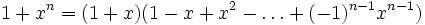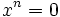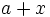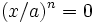# Invertible plus nilpotent implies invertible

View other indispensable lemmata

## Statement

In a commutative unital ring, the sum of an invertible element and a nilpotent element is an invertible element.

## Facts used$1 + x^n = (1 + x)(1 - x + x^2 - \ldots + (-1)^{n-1}x^{n-1})$

## Proof

Given: A commutative unital ring$A$, elements$a,x \in A$ such that$a$ is invertible, and$x^n = 0$ for some positive integer$n$

To prove:$a + x$ is invertible

Proof: Since$a$ is invertible, it suffices to prove that$1 + x/a$ is invertible. Since$x^n = 0$, we also have$(x/a)^n = 0$. The above formula then tells us that$1 + x/a$ is invertible, completing the proof.